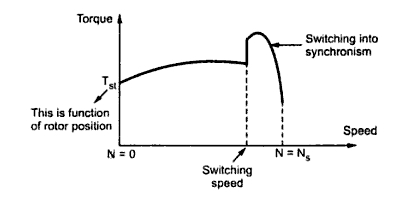### Reluctance Motors

The reluctance motor has basically two main parts called stator and rotor.
The stator has a laminated construction, made up of stampings. The stampings are slotted on its periphery to carry the winding called stator winding. The stator carries only one winding. This is excited by single phase a.c. supply. The laminated construction keeps iron losses to minimum. The stampings are made up of material like silicon steel which minimises the hysteresis loss. The stator winding is wound for certain definite number of poles.
The rotor has a particular shape. Due to its shape, the air gap between stator and rotor is not uniform. No d.c. supply is given to the rotor. The rotor is free to rotate. The reluctance i.e. resistance of magnetic circuits depends on the air gap. More the air gap, more the reluctance and viceversa. Due to variable air gap between stator and rotor, when rotor rotates, reluctance between stator and rotor also changes. The stator and rotor are designed in such a manner that the variation of the inductance of the windings is sinusoidal with respect to the rotor position.
The construction of the reluctance motor is shown in the Fig. 1(a) while the practical rotor of a reluctance motor is shown in the Fig. 1(b)Fig. 1  Reluctance motor

1.1 Working principle
The stator consists of a single winding called main winding. But single winding can not produce rotating magnetic field. So for production of rotating magnetic field, there must be at least two windings separated by certain phase angle. Hence stator consists of an additional winding called auxiliary winding which consists of capacitor in series with it. Thus there exists a phase difference between the currents carried by the two windings and corresponding fluxes. Such two fluxes react to produce the rotating magnetic field. The technique is called split phase technique of production of rotating magnetic field. The speed of this field is synchronous speed which is decided by the number of poles for which stator winding is wound.
The rotor carries the short circuited copper or aluminium bars and it acts as squirrel cage rotor of an induction motor. If an iron piece is placed in a magnetic field, it aligns itself in a minimum reluctance position and gets locked magnetically. Similarly in the reluctance motor, rotor tries to align itself with the axis of rotating magnetic field in a minimum reluctance position. But due to rotor inertia it is not possible when rotor is standstill. So rotor starts rotating near synchronous speed as a squirrel cage induction motor. When the rotor speed is about synchronous, stator magnetic field pulls rotor into synchronism i.e. minimum reluctance position and keeps it magnetically locked. The rotor continues to rotate with a speed equal to synchronous speed. Such a torque exerted on the rotor is called the reluctance torque. Thus finally the reluctance motor runs as a synchronous motor. The resistance of the rotor must be very small and the combined inertia of the rotor and the load should be small to run the motor as a synchronous motor.
1.2 Mathematical Analysis
Consider an elementary reluctance motor as shown in the Fig.2.Fig. 2 Elementary reluctance motor

The variation of the inductance of the windings is sinusoidal with respect to rotor position. The variation of the inductance with respect to θ is of double frequency and is given by,
L(θ) = L'' + L' cos2θ
The stator winding is excited by a.c. supply hence
i = Im sin ωt
The energy stored is a function of inductance and given by,
W = 0.5 L(θ)i2
The flux linkage is given by,
λ(θ) = L(θ)i
Then the torque is given by,

Substituting the values of i and L,

If rotor is rotating at an angular velocity  ωm then finally the torque equation can be expressed interms of ω and ωm as,
T = - 0.5 Im2 L' {sin2(ωm t- δ) - 0.5(sin2(ωm t + ωt- δ) + sin2(ωm t- ωt - δ)}
where       θ = ωm t - δ
and            δ = Rotor position at t = 0
The above equation gives instantaneous torque produced. The average torque is zero as average of each term in the above equation is zero. The value of torque is not zero when  ω = ωm and at this condition the magnitude of the average torque is,

The speed corresponding to the frequency ω = ωm is nothing but the synchronous speed. The δ is a torque angle. The maximum torque occurs at δ = 45which is termed as pull-out torque.
Note : Any load demanding torque more than pull-out torque pulls the motor out of synchronism.
1.3 Torque Speed Characteristics
The torque speed characteristic is shown in the Fig 3 The starting torque is highly dependent on the position of the rotor.Fig. 3 Torque speed characteristic of reluctance motor

The reluctance motor has following advantages,
1) No d.c. supply is necessary for rotor.
2) Constant speed characteristics.
3) Robust construction.
4) Less maintenance.
1.5 Laminations
The reluctance motor has following laminations,
1- Less efficiency
2- Poor power factor
3- Need of every low inertia motor
4- Less capacity to drive the loads.
1.6 Applications
This motor is used in signalling devices, control apparatus, automatic regulators, recording instruments, clocks and all kind of timing devices, teleprinters, gramophones etc.